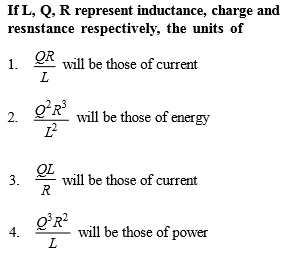The dimensional formula of the coefficient of thermal conductivity is :

1.  $\left[{\mathrm{M}}^{1}{\mathrm{L}}^{1}{\mathrm{T}}^{-3}{\mathrm{K}}^{-1}\right]$

2.  $\left[{\mathrm{M}}^{3}{\mathrm{L}}^{-1}{\mathrm{T}}^{-3}\mathrm{K}\right]$

3.  $\left[{\mathrm{M}}^{1}{\mathrm{L}}^{-3}{\mathrm{T}}^{-1}{\mathrm{K}}^{-1}\right]$

4.  $\left[{\mathrm{M}}^{1}{\mathrm{L}}^{1}{\mathrm{T}}^{-3}\mathrm{K}\right]$

Concept Questions :-

Dimensions
NEET 2021 - Achiever Batch - Aryan Raj Singh

Difficulty Level:[Only for Dropper batch]

Concept Questions :-

Dimensions
NEET 2021 - Achiever Batch - Aryan Raj Singh

Difficulty Level:

What are the dimensions of latent heat?

(1) $M{L}^{2}{T}^{-2}$

(2) $M{L}^{-2}{T}^{-2}$

(3) ${M}^{0}L{T}^{-2}$

(4) ${M}^{0}{L}^{2}{T}^{-2}$

Concept Questions :-

Dimensions
NEET 2021 - Achiever Batch - Aryan Raj Singh

Difficulty Level:

The quantity $\frac{1}{2}{\in }_{0}{E}^{2}$ has dimensional formula same as        [Only for dropper batch]

(1) $\frac{1}{2}C{V}^{2}$

(2) $\frac{1}{2}L{I}^{2}$

(3)

(4) Power

Concept Questions :-

Dimensions
NEET 2021 - Achiever Batch - Aryan Raj Singh

Difficulty Level:

Light year is a unit of

(1) Time

(2) Mass

(3) Distance

(4) Energy

Concept Questions :-

Dimensions
NEET 2021 - Achiever Batch - Aryan Raj Singh

Difficulty Level:

The magnitude of any physical quantity :

(1) Depends on the method of measurement

(2) Does not depend on the method of measurement

(3) Is more in SI system than in CGS system

(4) Directly proportional to the fundamental units of mass, length and time

Concept Questions :-

Dimensions
NEET 2021 - Achiever Batch - Aryan Raj Singh

Difficulty Level:

Which of the following is not equal to watt ?

(1) Joule/second

(2) Ampere $×$ volt

(3) (Ampere)2 $×$ ohm

(4) Ampere/volt

Concept Questions :-

Dimensions
NEET 2021 - Achiever Batch - Aryan Raj Singh

Difficulty Level:

Newton–second is the unit of

(1) Velocity

(2) Angular momentum

(3) Momentum

(4) Energy

Concept Questions :-

Dimensions
NEET 2021 - Achiever Batch - Aryan Raj Singh

Difficulty Level:

Which of the following is not represented in correct unit

(1) $\frac{\text{Stress}}{\text{Strain}}=N\text{​}/\text{​}{m}^{2}$

(2) Surface tension = $N\text{​}/\text{​}m$

(3) Energy $=kg\text{​-​}m\text{​}/\text{​}\mathrm{sec}$

(4) Pressure $=N\text{​}/\text{​}{m}^{2}$

Concept Questions :-

Dimensions
NEET 2021 - Achiever Batch - Aryan Raj Singh

Difficulty Level:

One second is equal to :

(1) 1650763.73 time periods of $Kr$ clock

(2) 652189.63 time periods of $Kr$ clock

(3) 1650763.73 time periods of $Cs$ clock

(4) 9192631770 time periods of $Cs$ clock

Concept Questions :-

Dimensions